﻿ Python: Find whether a given number is even or odd, print out an appropriate message to the user - w3resource# Python: Find whether a given number is even or odd, print out an appropriate message to the user

## Python Basic: Exercise-21 with Solution

Write a Python program to find whether a given number (accept from the user) is even or odd, print out an appropriate message to the user.

Pictorial Presentation of Even Numbers: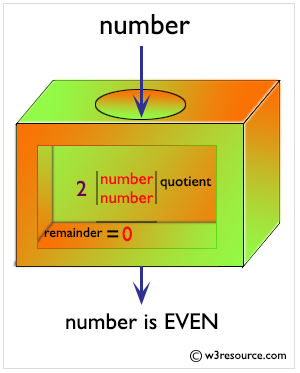Pictorial Presentation of Odd Numbers: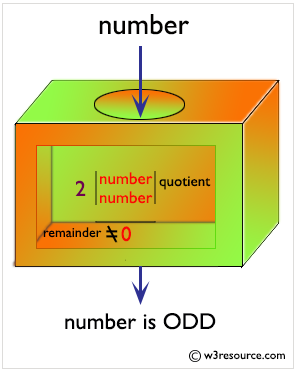Sample Solution:-

Python Code:

``````num = int(input("Enter a number: "))
mod = num % 2
if mod > 0:
print("This is an odd number.")
else:
print("This is an even number.")```
```

Sample Output:

```Enter a number: 5
This is an odd number.
```

Even Numbers between 1 to 100: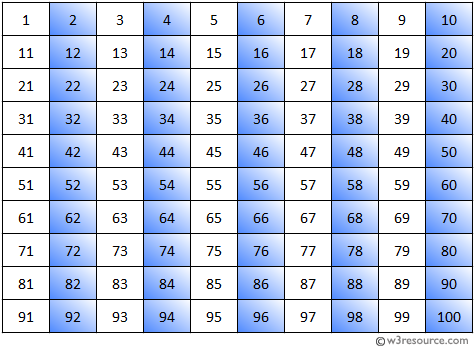Odd Numbers between 1 to 100: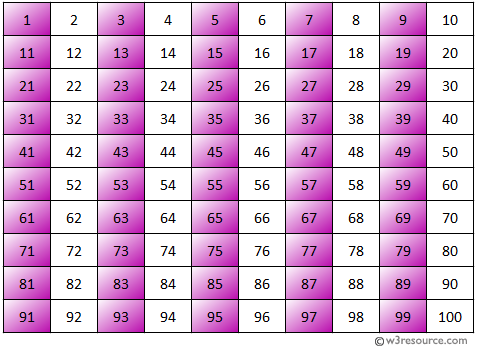Flowchart: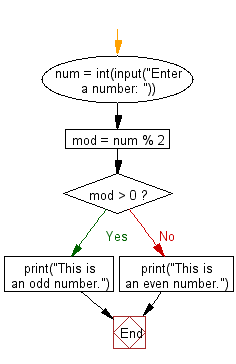## Visualize Python code execution:

The following tool visualize what the computer is doing step-by-step as it executes the said program:

Python Code Editor:

Have another way to solve this solution? Contribute your code (and comments) through Disqus.

What is the difficulty level of this exercise?

Test your Python skills with w3resource's quiz

﻿

## Python: Tips of the Day

Unpack variables from iterable:

```# One can unpack all iterables (tuples, list etc)
>>> a, b, c = 1, 2, 3
>>> a, b, c
(1, 2, 3)

>>> a, b, c = [1, 2, 3]
>>> a, b, c
(1, 2, 3)
```